## 解法一：暴力（遍历不同的高度）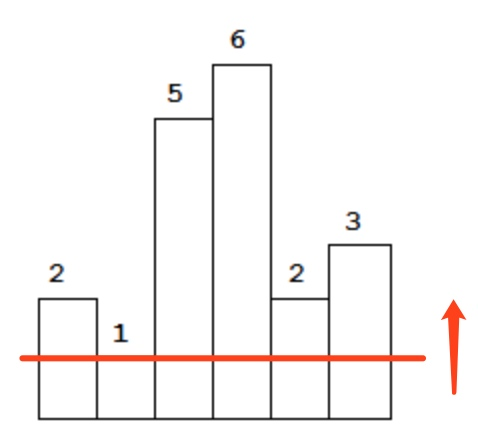func largestRectangleArea(heights []int) int {
res := 0
set := map[int]bool{}
for _, curHeight := range heights {
if _, ok := set[curHeight]; ok {
continue
}
set[curHeight] = true
preIndex := -1 // 前一个高度小于当前柱高 curHeight 的柱子下标
for i, h := range heights {
if h < curHeight {
// 在此时，i 是下一个高度小于当前柱高的柱子下标
// i-preIndex-1 即高度大于等于当前柱高的连续柱子个数
res = max(res, curHeight*(i-preIndex-1))
preIndex = i
}
i++
}
res = max(res, curHeight*(len(heights)-1-preIndex))
}
return res
}


## 解法二：分治

func largestRectangleArea(heights []int) int {
if len(heights) == 0 {
return 0
}
return dfs(heights, 0, len(heights)-1)
}

func dfs(heights []int, start, end int) int {
if start > end {
return -1
}
if start == end {
return heights[start]
}
minIndex := start
for i := start + 1; i <= end; i++ {
if heights[i] < heights[minIndex] {
minIndex = i
}
}
curMax := (end - start + 1) * heights[minIndex]
leftMax := dfs(heights, start, minIndex-1)
rightMax := dfs(heights, minIndex+1, end)
return max(curMax, max(leftMax, rightMax))
}


## 解法三：分治+线段树

func largestRectangleArea(heights []int) int {
if len(heights) == 0 {
return 0
}
root := BuildSegmentTree(heights, 0, len(heights)-1) // 1. 构建线段树
return dfs(root, heights, 0, len(heights)-1)
}

func dfs(sTree *SegmentTreeNode, heights []int, start, end int) int {
if start > end {
return -1
}
if start == end {
return heights[start]
}
minIndex := sTree.Query(heights, start, end) // 2. 使用 Query() 方法查找区间最小值的下标
curMax := (end - start + 1) * heights[minIndex]
leftMax := dfs(sTree, heights, start, minIndex-1)
rightMax := dfs(sTree, heights, minIndex+1, end)
return max(curMax, max(leftMax, rightMax))
}


type SegmentTreeNode struct {
start int
end   int
min   int // 本题线段树节点保存的不是区间最小值，而是最小值所在的下标
left  *SegmentTreeNode
right *SegmentTreeNode
}

func BuildSegmentTree(nums []int, left, right int) *SegmentTreeNode {
if left > right {
return nil
}
root := &SegmentTreeNode{left, right, left, nil, nil} // 根据节点区间的左边界值初始化
if left == right {
return root
}
mid := (left + right) / 2
root.left = BuildSegmentTree(nums, left, mid)
root.right = BuildSegmentTree(nums, mid+1, right)
if nums[root.left.min] < nums[root.right.min] {
root.min = root.left.min
} else {
root.min = root.right.min
}
return root
}

func (root *SegmentTreeNode) Query(nums []int, start, end int) int {
if start > root.end || end < root.start {
return -1
}
if start <= root.start && end >= root.end {
return root.min
}
leftMin := root.left.Query(nums, start, end)
rightMin := root.right.Query(nums, start, end)
if leftMin < 0 {
return rightMin
}
if rightMin < 0 {
return leftMin
}
if nums[leftMin] < nums[rightMin] {
return leftMin
}
return rightMin
}

func max(a, b int) int {
if a > b {
return a
}
return b
}


## 解法四：暴力（遍历不同的柱子）func largestRectangleArea(heights []int) int {
res := 0
for i, h := range heights {
left, right := i, i
for left > 0 && heights[left-1] >= h {
left--
}
for right < len(heights)-1 && heights[right+1] >= h {
right++
}
res = max(res, h*(right-left+1))
}
return res
}


## 解法五：单调栈

heights[i] × (right-left-1) // 其中 i=stack[top], left=stack[top-1], right=j


[2, 1, 5, 6, 2, 3] 为例，过程如下：

1. 初始化：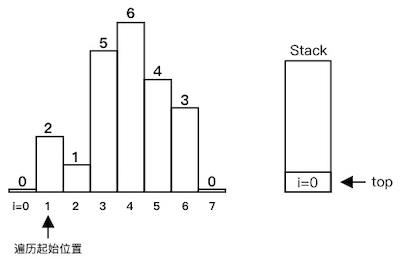2. 开始遍历。heights > heights[stack[top]=0]，入栈：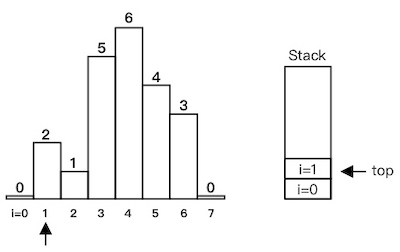3. heights < heights，出栈，计算红色部分面积，然后 i=2 入栈。可以看到，红色部分正好位于栈顶下一个元素与当前遍历元素之间，下同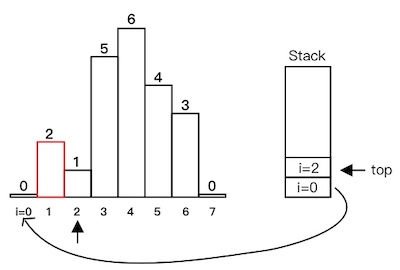4. i=3i=4 依次入栈：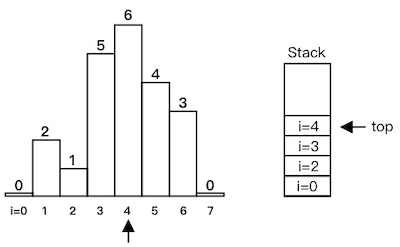5. heights < heights，出栈，计算红色部分面积：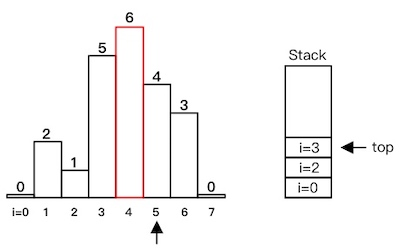6. heights < heights，出栈，计算红色部分面积，然后 i=5 入栈：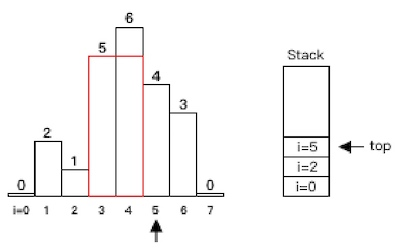7. heights < heights，出栈，计算红色部分面积：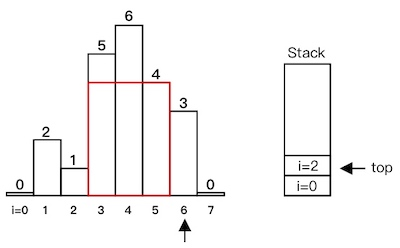8. i=6 入栈：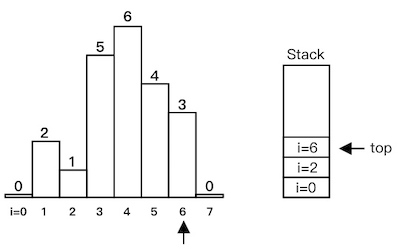9. heights < heights，出栈，计算红色部分面积：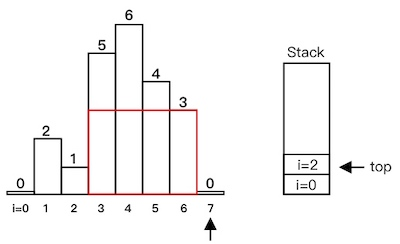10. heights < heights，出栈，计算红色部分面积：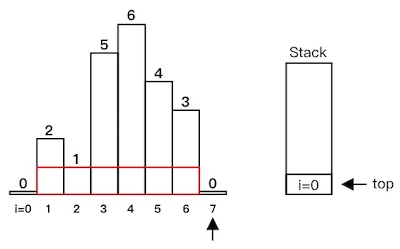11. 遍历结束，输出计算过的最大面积

func largestRectangleArea(heights []int) int {
res := 0
heights = append([]int{0}, heights...)
heights = append(heights, 0) // 首尾添加 0
stack := NewStack()
stack.Push(0)                       // 下标 0 入栈
for i := 1; i < len(heights); i++ { // 从 1 开始遍历
for heights[i] < heights[stack.Top()] {
h := heights[stack.Pop()]
left, right := stack.Top(), i
res = max(res, h*(right-left-1))
}
stack.Push(i)
}
return res
}

// 以下是栈的模板代码

type ElementType = int
type Stack struct {
s []ElementType
}

func NewStack() *Stack {
return &Stack{
s: make([]ElementType, 0),
}
}

func (s *Stack) Empty() bool {
return len(s.s) == 0
}

func (s *Stack) Top() ElementType {
if s.Empty() {
return 0
}
return s.s[len(s.s)-1]
}

func (s *Stack) Pop() ElementType {
if s.Empty() {
return 0
}
t := s.s[len(s.s)-1]
s.s = s.s[:len(s.s)-1]
return t
}

func (s *Stack) Push(v ElementType) {
s.s = append(s.s, v)
}